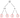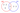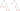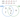## Prime Factors, HCF and LCM

Understanding prime factors is important to be able to find the Lowest Common Multiple (LCM) and Highest Common Factor (HCF) of two or more numbers. Make sure you are happy with the following topics before continuing.

KS3Level 4-5

## Prime Factorisation and Factor Trees

We define a prime factor of any given number to be any factor that the number has, that is also a prime number.

Every positive whole number has a unique prime factorisation – a list of prime numbers that, when multiplied together, give you the original number. In more complicated cases, we use something called a factor tree.

Example: Determine the prime factorisation of $60$.

Step 1: To construct a factor tree, think of $2$ numbers which multiply together to make $60$ – here, we’ve gone with $10$ and $6$.

Step 2: Draw two branches coming down from $60$, and at the end of the branches write the two factors that you chose.

Step 3: If a factor is prime, then  circle it. If a factor is not prime, then repeat the process as shown in the factor tree below.

Step 4: The prime factorisation of $60$ is therefore

$60 = 2 \times 2 \times 3 \times 5$Step 5: We write this prime factorisation in index form, where if there is more than one of the same factor, we write it as a power instead, where the power is the number of times it occurs. So

$60= 2^2 \times 3\times 5$

KS3Level 4-5

## Highest Common Factor – HCF

The Highest Common Factor, or HCF, of two numbers is the biggest number that goes into both of them.

Example: Consider the numbers $12$ and $20$

The factors of $12$ are: $1$, $2$, $3$, $4$, $6$, and $12$

The factors of $20$ are: $1$, $2$, $4$, $5$, $10$, and $20$

They have a few factors in common, but the biggest factor they have in common is $4$, therefore $4$ is the HCF of $12$ and $20$.

KS3Level 4-5

## Lowest Common Multiple – LCM

The lowest common multiple, or LCM, of two numbers is the smallest number that is a multiple of both of them.

Example: Consider the numbers $5$ and $7$

Multiples of $5$ are: $5$, $10$, $15$, $20$, $25$, $30$, $35$, $40$, $45$, …

Multiples of $7$ are: $7$, $14$, $21$, $28$, $35$, $42$, … and so on.

So, we can see that the first occurrence of a number which is a multiple of both of these numbers is $35$, therefore $35$ is the LCM of $5$ and $7$.

KS3Level 4-5
KS3Level 4-5

## HCF and LCM – Venn Diagrams

For large numbers, the easiest way to find the HCF and LCM is to use Venn diagrams

Example: Find the HCF and LCM of $60$ and $27$.

Step 1: We first need the prime factorisation of both numbers, in which we would use factor trees. However we already have the prime factorisation of $60$, which is

$60 = 2 \times 2 \times 3 \times 5 = 2^2 \times 3 \times 5$  and $27= 3 \times 3 \times 3 = 3^3$Step 2: Now, we draw a Venn diagram where one circle is for prime factors of $60$ and one circle is for prime factors of $27$.

Step 3: Looking at the list of factors, if one is shared by both numbers, then we will put it in the intersection and cross it off both lists.

$\textcolor{red}{60} = 2 \times 2 \times \bcancel{3} \times 5$  and  $\textcolor{blue}{ 27} = \bcancel{3} \times 3 \times 3$

Step 4: Any factors that are not shared and haven’t been crossed out, we put in their respective circles.

Step 5: To find the HCF, we multiply the numbers in the intersection (these are the factors that are common between both numbers). Here there is only one number, so

HCF $= 3$

Step 6: To find the LCM, we multiply all of the numbers in the Venn diagram together. So

LCM $= 2 \times 2 \times 5 \times 3 \times 3 \times 3$

KS3Level 4-5
KS3Level 4-5

## Example: Prime Factor Tree

Find the LCM and HCF of $420$ and $132$.

[4 marks]

To do this method, we require the full prime factorisation of both $420$ and $132$. So, we’re going to use the factor tree method.

The prime factor tree for $420$ can be seen on the right,

This gives,

$2\times2\times3\times 5\times 7 = 2^2 \times 3 \times 5 \times 7$

Going through the same process, we get that the full prime factorisation of $132$ is

$2\times2\times 3\times 11 = 2^2 \times 3 \times 11$So, now that we have the prime factorisation, we need to draw a Venn diagram where one circle is for prime factors of $420$ and one circle is for prime factors of $132$.

Looking at the lists of factors, if one is shared by both numbers, then we will put it in the intersection and cross it off both lists.

Then, any factors that aren’t shared, and so haven’t been crossed out, will be put in their respective circles.

To find the HCF is to multiply the numbers in the intersection:

HCF $=2\times2\times3=12$

To find the LCM, all we need to do is multiply all the numbers now in the Venn diagram together:

LCM $=5\times7\times2\times 2\times3\times11=4620$KS3Level 4-5

### Take an Online Exam

#### Prime Factors LCM HCF Online Exam

Example Questions

Question 1: Find the unique prime factorisation of $72$.

Write your answer using index notation.

[2 marks]

KS3Level 4-5

The prime factors of a number can be displayed using a prime factor tree.

The prime factorisation of $72$ is,$72=2\times2\times2\times3\times3$

Written in index notation, the answer is,

$72=2^3\times3^2$

Question 2: Find the prime factorisation of $140$.

Write your answer using index notation.

[2 marks]

KS3Level 4-5

The prime factors of a number can be displayed using a prime factor tree.

The prime factorisation of $140$ is,$140=2\times2\times5\times7$

Written in index notation, the answer is,

$140=2^2\times5\times7$

Question 3: Find the HCF and LCM of $24$ and $40$.

[4 marks]

KS3Level 4-5

First, we have to find the prime factorisation of $24$ and of $40$:

Prime factors of $24$$2\times2\times2\times3$

Prime factors of $40$: $2\times2\times2\times5$

To find the HCF, find any prime factors that are in common between both numbers.

HCF$2\times2\times2=8$

Next, cross any numbers used so far off from the products.

Prime factors of $24$$\cancel{2}\times\cancel{2}\times\cancel{2}\times3$

Prime factors of $40$: $\cancel{2}\times\cancel{2}\times\cancel{2}\times5$

To find the LCM, multiply the HCF by all the factors that have not been crossed out so far.

LCM = $8\times3\times5=120$

Question 4: Find the HCF and LCM of $495$ and $220$.

[4 marks]

KS3Level 4-5

The prime factors of both $495$ and $220$ can be displayed using prime factor trees.So, the factorisation of $220$ is,

$220=2\times2\times5\times11$

and the factorisation of $495$ is,

$495=3\times3\times5\times11$

Now, we will draw a Venn diagram with one circle containing the factors of $495$ and the other containing the factors of $220$. Any prime factors shared by these two numbers are to be placed in the intersection.

$495=3\times3\times\cancel5\times\cancel11$

$220=2\times2\times\cancel5\times\cancel11$The HCF can be calculated by multiplying the numbers in the intersection together,

$\text{HCF }=5\times11=55$

Finally, we find the LCM by multiplying all the numbers in the Venn diagram together,

$\text{LCM }=3\times3\times5\times11\times2\times2=1,980$

Question 5: Find the HCF and LCM of $32$, $152$ and $600$.

[5 marks]

KS3Level 4-5

First, we have to find the prime factorisation of $32$, $152$ and of $600$:

Prime factors of $32=$$2\times2\times2\times2\times2$

Prime factors of $152=$ $2\times2\times2\times19$

Prime factors of $600=$ $2\times2\times2\times3\times5\times5$

Then we can place each prime factor in the correct circle in the Venn diagram. Any common factors should be placed in the intersections of the circles.The highest common factor (HCF) is found by multiplying together the numbers in the intersection of all three of the circles.

HCF$2\times2\times2=8$

The lowest common multiple (LCM) is found by multiplying together the numbers from all sections of the circles.

LCM$2\times2\times2\times2\times2\times3\times5\times5\times19=45600$

### Worksheets and Exam Questions

(NEW) Prime Factors, HCF, LCM - Exam Style Questions - MMELevel 4-5NewOfficial MME

### Drill Questions

#### Factors Multiples Primes - Drill Questions

By clicking continue and using our website you are consenting to our use of cookies in accordance with our Cookie Policy